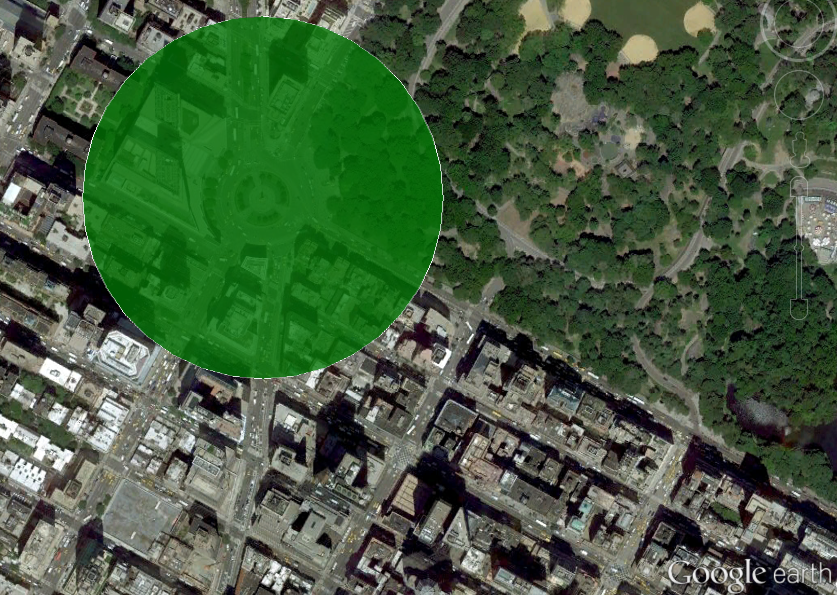# Getting started¶

## Installing¶

```pip install polycircles
```

Generates a circle approximation readable by simpleKML.

```from polycircles import polycircles

polycircle = polycircles.Polycircle(latitude=40.768085,
longitude=-73.981885,
number_of_vertices=36)
kml = simplekml.Kml()
pol = kml.newpolygon(name="Columbus Circle, Manhattan",
outerboundaryis=polycircle.to_kml())
pol.style.polystyle.color = \
simplekml.Color.changealphaint(200, simplekml.Color.green)
kml.save("test_kml_polygon_3_manhattan.kml")
```

Note that a polygon with 36 vertices looks pretty much like a circle:A Polycircle above Columbus Square, Columbus Square, Manhattan, NYC, United States

## Sequence of lat-lon points¶

`polycircles` can simply generate a series of lat-lon tuples, for any non-KML usage.

```   import pprint
from polycircles import polycircles
polycircle = polycircles.Polycircle(latitude=32.074523,
longitude=34.791469,